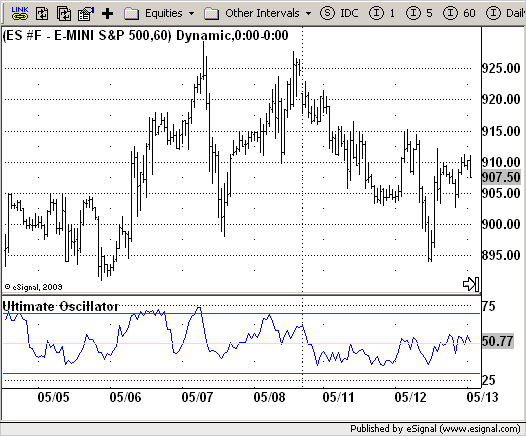# Ultimate Oscillator

ICE Data Services -

UltimateOscillator.efs

File Name: UltimateOscillator.efs

Description:
Ultimate Oscillator

Formula Parameters:

• Short Period : 7
• Med Period : 14
• Long Period : 28

Notes:
Oscillators typically compare a security's smoothed price with its price x-periods ago. Larry Williams noted that the value of this type of oscillator can vary greatly depending on the number of time periods used during the calculation. Thus, he developed the Ultimate Oscillator that uses weighted sums of three oscillators, each of which uses a different time period.
The three oscillators are based on Williams' definitions of buying and selling "pressure".

UltimateOscillator.efsEFS Code:

```/*********************************
Provided By:
eSignal (Copyright c eSignal), a division of Interactive Data
Formula Script (EFS) is for educational purposes only and may be
modified and saved under a new file name.  eSignal is not responsible
for the functionality once modified.  eSignal reserves the right
to modify and overwrite this EFS file with each new release.

Description:
Ultimate Oscillator

Version:            1.0  05/08/2009

Formula Parameters:                     Default:
Short Period                        7
Med Period                          14
Long Period                         28

Notes:
Oscillators typically compare a security's smoothed price with its
price x-periods ago. Larry Williams noted that the value of this type
of oscillator can vary greatly depending on the number of time periods
used during the calculation. Thus, he developed the Ultimate Oscillator
that uses weighted sums of three oscillators, each of which uses a different
time period.
The three oscillators are based on Williams' definitions of buying and
selling "pressure."
**********************************/
var fpArray = new Array();
var bInit = false;

function preMain() {
setStudyTitle("Ultimate Oscillator");
setCursorLabelName("UO");
setStudyMax(80);
setStudyMin(20);
var x = 0;
fpArray[x] = new FunctionParameter("Period_short", FunctionParameter.NUMBER);
with(fpArray[x++]) {
setName("Short Period");
setLowerLimit(1);
setDefault(7);
}
fpArray[x] = new FunctionParameter("Period_med", FunctionParameter.NUMBER);
with(fpArray[x++]) {
setName("Med. Period");
setLowerLimit(1);
setDefault(14);
}
fpArray[x] = new FunctionParameter("Period_long", FunctionParameter.NUMBER);
with(fpArray[x++]) {
setName("Long Period");
setLowerLimit(1);
setDefault(28);
}
}

var xUO = null;

function main(Period_short, Period_med, Period_long){
var nBarState = getBarState();
if (nBarState == BARSTATE_ALLBARS) {
if (Period_short == null) Period_short = 7;
if (Period_med == null) Period_med = 14;
if (Period_long == null) Period_long = 28;
}
if (bInit == false) {
xUO = efsInternal("Calc_UO", Period_short, Period_med, Period_long);
bInit =  true;
}
nUO = xUO.getValue(0);
if (nUO == null) return;
return nUO;
}

var xMA1TL = null;
var xMA2TL = null;
var xMA3TL = null;
var xMA1TR = null;
var xMA2TR = null;
var xMA3TR = null;
var xTL = null;
var xTR = null;
var bSecondInit = false;

function Calc_UO(Period_short, Period_med, Period_long) {
var nMA1TL = 0;
var nMA2TL = 0;
var nMA3TL = 0;
var nMA1TR = 0;
var nMA2TR = 0;
var nMA3TR = 0;
var nRes = 0;
if (bSecondInit == false) {
xTL = efsInternal("Calc_TrueLow");
xMA1TL = sma(Period_short, xTL);
xMA2TL = sma(Period_med, xTL);
xMA3TL = sma(Period_long, xTL);
xTR = atr(1);
xMA1TR = sma(Period_short, xTR);
xMA2TR = sma(Period_med, xTR);
xMA3TR = sma(Period_long, xTR);
bSecondInit = true;
}
nMA1TL = xMA1TL.getValue(0);
nMA2TL = xMA2TL.getValue(0);
nMA3TL = xMA3TL.getValue(0);
nMA1TR = xMA1TR.getValue(0);
nMA2TR = xMA2TR.getValue(0);
nMA3TR = xMA3TR.getValue(0);
if (nMA1TL == null || nMA2TL == null || nMA3TL == null) return;
nRes = (4 * nMA1TL / nMA1TR + 2 * nMA2TL / nMA2TR + nMA2TL / nMA2TR) / 7;
return nRes * 100;
}

function Calc_TrueLow() {
var nRes = 0;
var nClose1 = close(-1);
if (nClose1 == null) return;
nRes = Math.min(low(0), nClose1);
nRes = close(0) - nRes;
return nRes;
}```